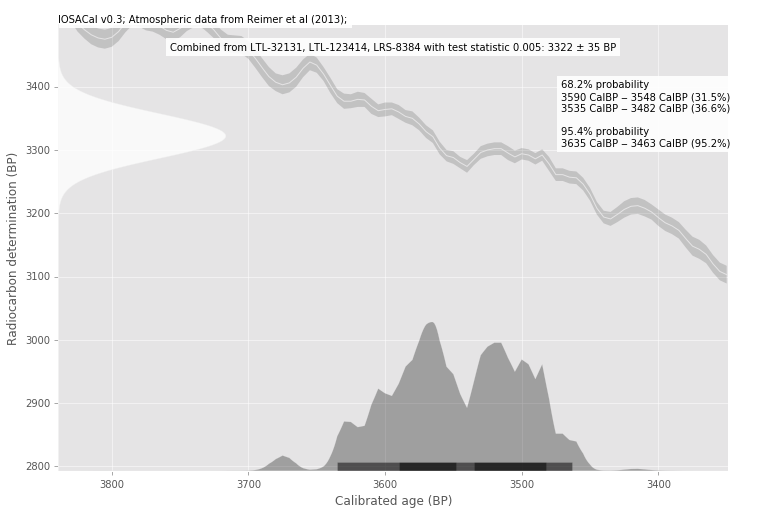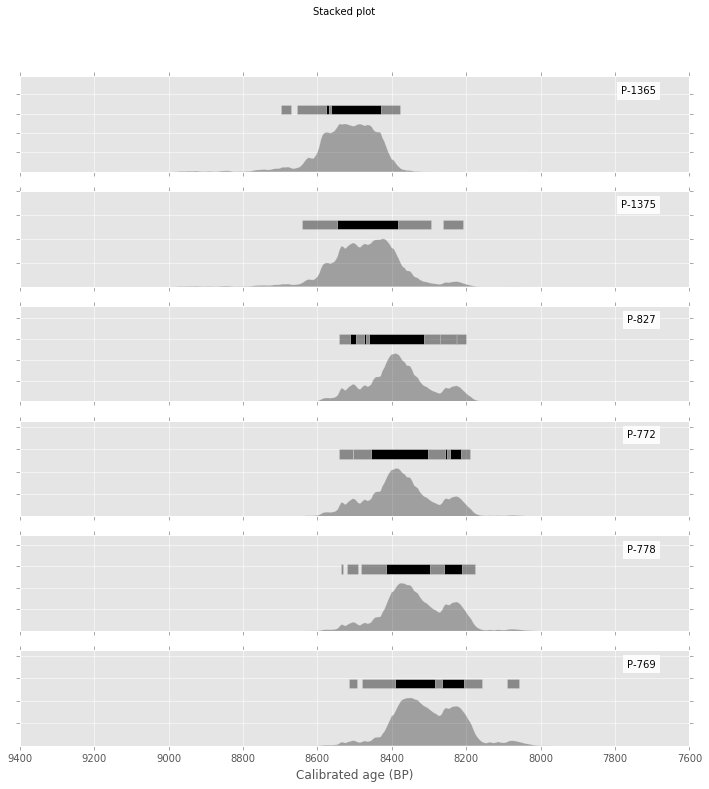# IOSACal in action!¶

IOSACAL is the radiocarbon calibration software from the IOSA project. It's written in Python and it's open source. This demo is based on the current version (0.3). The documentation is at http://c14.iosa.it/.

An interactive session with IOSACal in Jupyter Notebook is the best way to get familiar with the software and create beautiful reports that are also self-explaining. Reproducible science!

This is the standard import needed to start working with IOSACal and Jupyter Notebook:

In :
from iosacal import R, combine, iplot
%matplotlib inline


If you are going to define labels in languages different from English, please do this:

In :
from __future__ import unicode_literals


Now define three radiocarbon determinations and combine them:

In :
rs = [R(3320, 65, 'LTL-32131'), R(3320,65,'LTL-123414'), R(3325,55,'LRS-8384')]
cr = combine(rs)
print(cr)

RadiocarbonSample( Combined from LTL-32131, LTL-123414, LRS-8384 with test statistic 0.005 : 3322 ± 35 )


Now that we have a combined age, calibrate it using the IntCal13 curve:

In :
calcr = cr.calibrate('intcal13')


The calibrated date can be plotted as normal:

In :
iplot(calcr)## Multiple dates¶

IOSACal can plot multiple dates for comparing, and you can use Python as normal

In :
rs = [R(7729, 80, "P-1365"),
R(7661, 99, "P-1375"),
R(7579, 86, "P-827"),
R(7572, 92, "P-772"),
R(7538, 89, "P-778"),
R(7505, 93, "P-769")]
multiple = [r.calibrate('intcal13') for r in rs]


It's also possible to change Matplotlib settings without need to edit the underlying source code:

In :
import matplotlib.pyplot as plt
plt.style.use('ggplot')


And create the resulting plot:

In :
iplot(multiple)Any plot can be saved directly to a file on disk, if needed:

In :
iplot(multiple, output="Catalhöyük East level VI A.pdf")This was a quick tour of IOSACal used interactively in Jupyter. Thank you!